EnchantedLearning.com is a user-supported site.
As a bonus, site members have access to a banner-ad-free version of the site, with print-friendly pages.

 +, - EnchantedLearning.comMath Dictionary x, ÷
 A B C D E F G H I J K L M N O P Q R S T U V W X Y ZS is for ...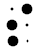scalarA scalar is a number (a magnitude) without a direction (compare with vector). For example, speed is a scalar; it tells you how fast something is traveling but not the direction.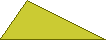scalene triangleThe sides of a scalene triangle are all different lengths.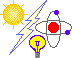scienceScientists study science to learn about the physical world. scientific notationScientific notation is a mathematical format used to write very large and very small numbers; this system avoids using a lot of zeros by using powers (exponents). In scientific notation, there is a number between 1 and 10 multiplied by a power of ten. For example, the number 250 written in scientific notation is 2.5 x 102. For another example, the number 0.000052 written in scientific notation is 5.2 x 10-5.
 secantSecant (abbreviated sec) is a trigonometric ratio, corresponding to the length of the hypotenuse divided by the length of the adjacent side of the right triangle. Secant is equal to 1/cosine. secant lineA secant line is a line that intersects a circle or curve in two places.secondThe second item comes after the first item and before the third item. Second is abbreviated 2nd. secondA second is a measure of angle that is equal to 1/60th of a minute (a minute is 1/60th of a degree).
 secondA second is a measure of time that is equal to 1/60th of a minute.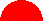semicircleA semicircle is half a circle.semi-major axisThe semi-major axis of an ellipse (a flattened circle) is half the length of the line segment across the longest part of the ellipse.semi-minor axisThe semi-minor axis of an ellipse (a flattened circle) is half the length of the line segment across the shortest part of the ellipse.
 1+1/2+1/3+1/4+1/5+...seriesA series is a sum of a sequence of numbers. setA set is a collection of numbers or objects. The items in a set are called the elements or members of that set. The empty set {} is also called the null set.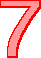sevenSeven is the number between six and eight. There are seven days in the week. A heptagon is a seven-sided figure.
 sineSine (abbreviated sin) is a trigonometric ratio, corresponding to the length of the opposite side divided by the length of the hypotenuse of the right triangle.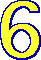sixSix is the number between five and seven. A six-sided figure is called a hexagon. Insects have six legs. skewTwo lines are skew if they do not lie on the same plane and are not parallel. Skew lines do not ever intersect. skip countingSkip counting is counting while skipping some numbers, like 2, 4, 6, 8, ....
 slopeThe slope of a line (also called the rise over the run) is the steepness of a line. To find the slope of a line, look at any two points on the line, (x1, y1) and (x2, y2) and determine the rise/run, or (y2-y1)/(x2-x1). When a linear equation is in the form: y=mx+b, m is the slope of the line (and b is the y-intercept). solveWhen you solve an equation or a problem, you find solutions for it.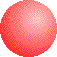sphereA sphere is a ball-shaped object. The volume of a sphere is 4πr3/3 (where r=radius of sphere).spiralA spiral is a curve that winds in on itself. Snail shells have a spiral pattern.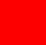squareA square is a four-sided figure (a quadrilateral) that has four, equally long sides which are at right angles to each other. square numbersSquare numbers are numbers that are the square of some integer. (The square of a number is that number times itself.) Examples are: 1 (which is 12), 4 (which is 22), 9 (which is 32), 16 (which is 42), 25 (which is 52), etc. square rootThe square roots of a number n are the numbers s such that s2=n. For example, the square roots of 4 are 2 and -2; the square roots of 9 are 3 and -3.starA star is a shape that has many points.subtendTo subtend is to be opposite to and to delimit. For example, in the triangle above, the side labeled b subtends the angle B. 1-1=05-3=2subtractionSubtraction is an operation in which one number is taken away from another number. For example, 4 - 1 = 3 (four minus one equals three). A number minus itself is equal to zero (for example, 3 - 3 = 0). Subtraction is the inverse operation of addition. That is, a + b - b = a. Subtraction is considered to be a binary operation because it is performed on two numbers.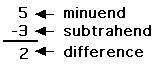subtrahendThe subtrahend is the number that is subtracted from another number in a subtraction problem.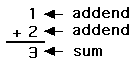sumThe sum is the answer to an addition problem.
 xsuperscriptsuperscriptA superscript is a raised text, usually in a smaller font. A superscript in mathematics often means a power or exponent. For example, x2 means x squared (or x to the second power or x times x). x0.5 means the square root of x. supplementary angleA supplementary angle is an angle that, when added to another angle, equals 180 degrees. ! /,< +-=÷¢symbolA symbol is a mark or sign that stands for something else. For example, the symbol ÷ means divide.symmetryA figure exhibits symmetry when part of the figure is the mirror image of another part of the figure. For example, an object has line (or reflection) symmetry when one side of the figure is a mirror image of the other across the line of symmetry (for example, a heart has linear symmetry). An object has plane symmetry when two halves of the object are mirror reflections of each other across a plane of symmetry (for example, a cylinder has plane symmetry). An object has radial (or point) symmetry when it is symmetric around a point (for example, a circle has point symmetry since every point on the circle has a mirror image of itself across the central point). x + y = 3x - y = 1system of equationsA system of equations is two or more independent equations that are solved together. For example, the system of equations: x + y = 3 and x - y = 1 has a solution of x=2 and y=1.

 +, - EnchantedLearning.comMath Dictionary x, ÷
 A B C D E F G H I J K L M N O P Q R S T U V W X Y Z
 Number Line Fractions Decimals Graphs Measurement Rounding

Click on an underlined word for more information on that subject. If the math term you are looking for is not in the dictionary, please e-mail us.

Enchanted Learning®
Over 35,000 Web Pages
Sample Pages for Prospective Subscribers, or click below

 Overview of Site What's New Enchanted Learning Home Monthly Activity Calendar Books to Print Site Index K-3 Crafts K-3 Themes Little ExplorersPicture dictionary PreK/K Activities Rebus Rhymes Stories Writing Cloze Activities Essay Topics Newspaper Writing Activities Parts of Speech Fiction The Test of Time Biology Animal Printouts Biology Label Printouts Biomes Birds Butterflies Dinosaurs Food Chain Human Anatomy Mammals Plants Rainforests Sharks Whales Physical Sciences: K-12 Astronomy The Earth Geology Hurricanes Landforms Oceans Tsunami Volcano Languages Dutch French German Italian Japanese (Romaji) Portuguese Spanish Swedish Geography/History Explorers Flags Geography Inventors US History Other Topics Art and Artists Calendars College Finder Crafts Graphic Organizers Label Me! Printouts Math Music Word Wheels

## Enchanted Learning Search

 Search the Enchanted Learning website for: